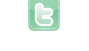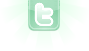## Using a variable in place of a class name in a method

I'm trying to write a method that can quickly add a given amount of objects from a given class on random free spaces on the map. For this, I've come up with the following code:
```public void place (int amount, Class objectType)
{
for (int i = 0; i < amount; i++)
{
objectType placeThis = new objectType;   //This is the problematic line
int x, y;
do {
x = Greenfoot.getRandomNumber (getWidth());
y = Greenfoot.getRandomNumber (getHeight());
} while (getObjectsAt (x, y, Actor.class).size() != 0);
```Actor placeThis = null;